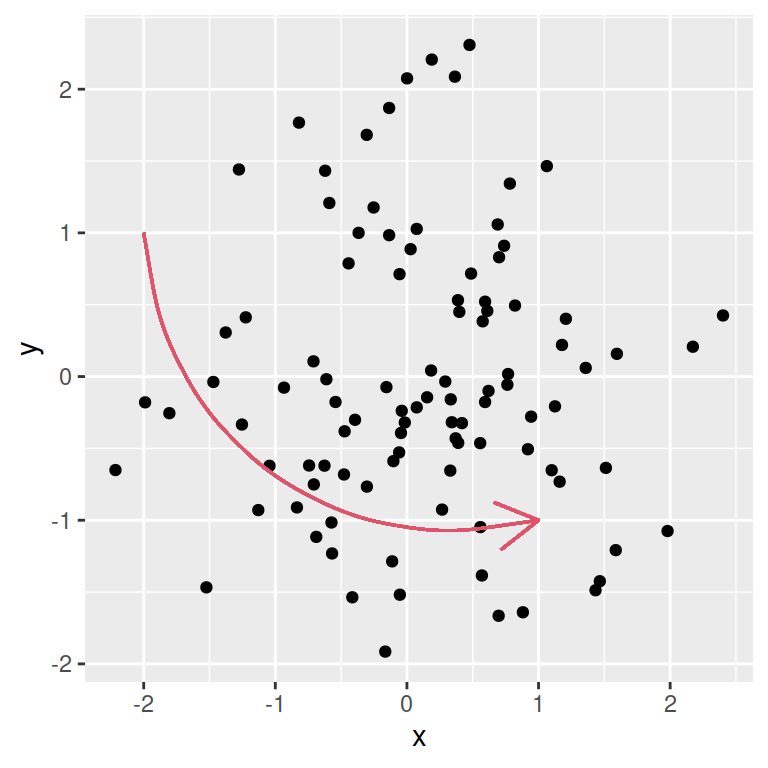# Reference lines, segments, curves and arrows in ggplot2

The `ggplot2` package has several functions to add annotation layers to the plots such as reference lines (`geom_vline`, `geom_hline` and `geom_abline`), segments (`geom_segment`), curves (`geom_curve`) and arrows (`arrows`). In this tutorial we are going to review the most common use cases of these functions.

## Vertical lines with `geom_vline`

Considering that you have a plot made with ggplot2 you can add a new layer with `geom_vline` to add a single or multiple vertical lines. You just need to pass a single value or a numeric vector to the `xintercept` argument of the function where you want the lines to be displayed.

Note that you can also customize graphical arguments such as `linetype`, `color` or `lwd`.

``````# install.packages("ggplot2")
library(ggplot2)

# Data
set.seed(1)
df <- data.frame(x = rnorm(100),
y = rnorm(100))

ggplot(df, aes(x = x, y = y)) +
geom_point() +
geom_vline(xintercept = 0,
linetype = 2,
color = 2)``````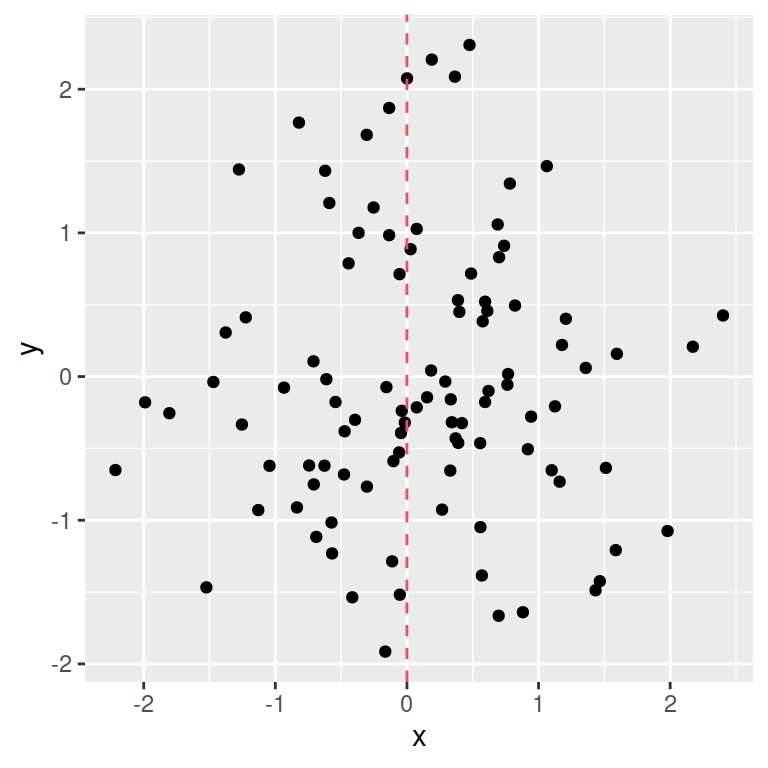Note that all functions of this tutorial allow doing this.

``````# install.packages("ggplot2")
library(ggplot2)

# Data
set.seed(1)
df <- data.frame(x = rnorm(100),
y = rnorm(100))

ggplot(df, aes(x = x, y = y)) +
geom_point() +
geom_vline(xintercept = -1:1,
linetype = 1,
color = 2:4)``````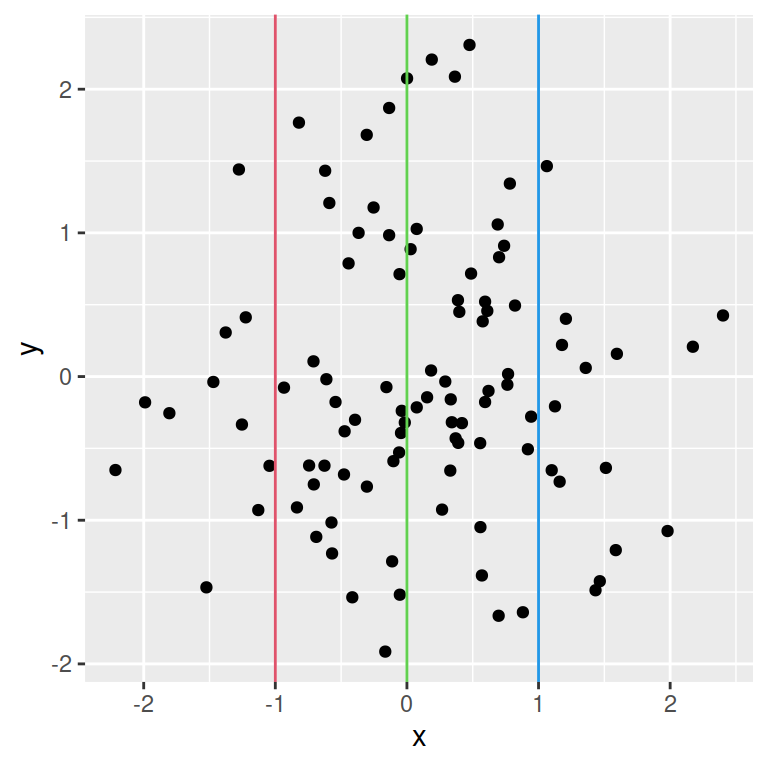## Horizontal lines with `geom_hline`

The `geom_hline` function behaves the same way as `geom_vline`. The only difference is that this function will add horizontal lines, so you will need to specify the `yintercept` argument instead of `xintercept`.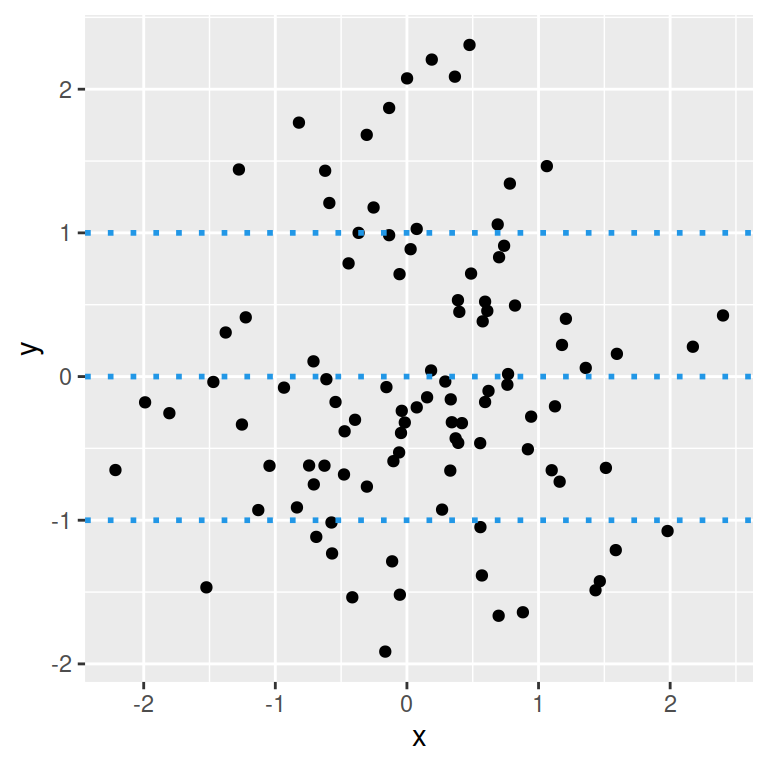``````# install.packages("ggplot2")
library(ggplot2)

# Data
set.seed(1)
df <- data.frame(x = rnorm(100),
y = rnorm(100))

ggplot(df, aes(x = x, y = y)) +
geom_point() +
geom_hline(yintercept = -1:1,
linetype = 3,
color = 4,
lwd = 1)``````

## Diagonal lines with `geom_abline`

The `geom_abline` function allows adding diagonal lines based on a intercept and a slope. The following example shows a diagonal line with intercept 0 and slope of 1.

``````# install.packages("ggplot2")
library(ggplot2)

# Data
set.seed(1)
df <- data.frame(x = rnorm(100),
y = rnorm(100))

ggplot(df, aes(x = x, y = y)) +
geom_point() +
geom_abline(intercept = 0,
slope = 1)``````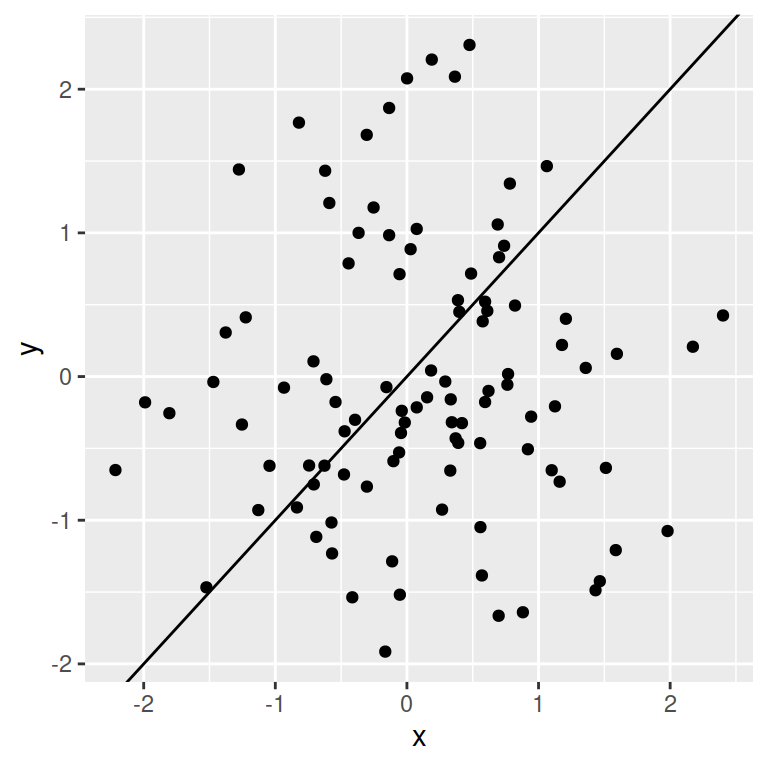## Segments with `geom_segment`

The previous functions can be used to add lines to the plots, but you cannot create segments with them. For that reason there exists the `geom_segment` function, which allows specifying the X and Y coordinates of the start and end of the desired segment with `x`, `y` (start) and `xend`, `yend` (end), respectively.

Note that if you pass the `arrow` function to the `arrow` argument you can create an arrow. See the arguments of the `arrow` function for further customization.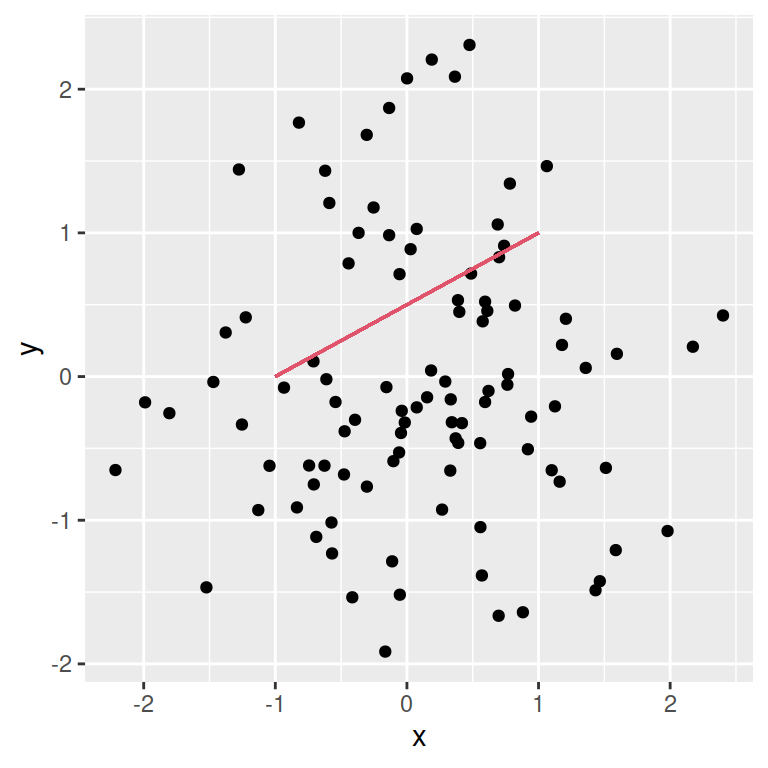``````# install.packages("ggplot2")
library(ggplot2)

# Data
set.seed(1)
df <- data.frame(x = rnorm(100),
y = rnorm(100))

ggplot(df, aes(x = x, y = y)) +
geom_point() +
geom_segment(x = -1, y = 0,
xend = 1, yend = 1,
color = 2)``````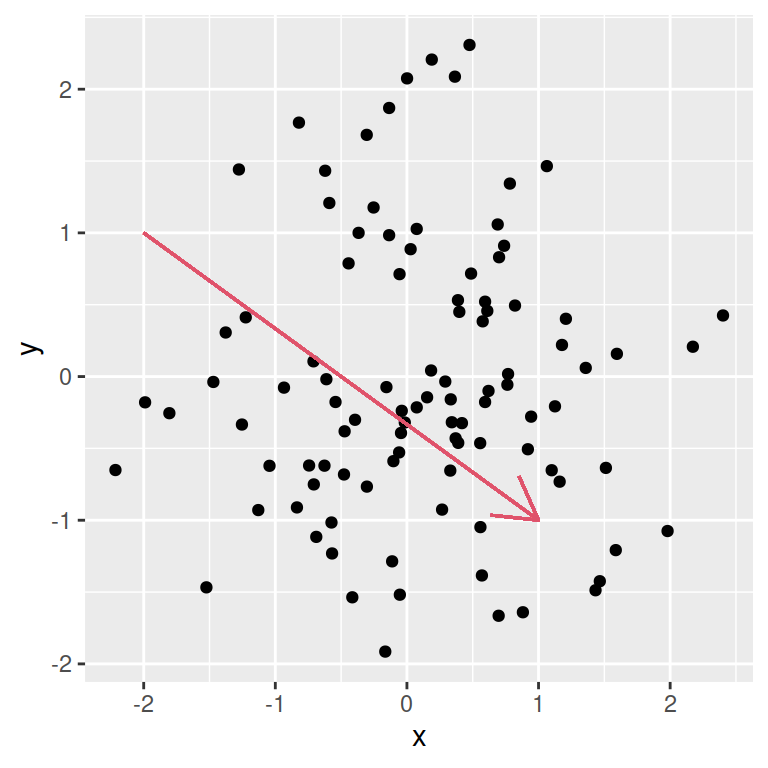Annotate arrow

``````# install.packages("ggplot2")
library(ggplot2)

# Data
set.seed(1)
df <- data.frame(x = rnorm(100),
y = rnorm(100))

ggplot(df, aes(x = x, y = y)) +
geom_point() +
geom_segment(x = -2, y = 1,
xend = 1, yend = -1,
color = 2,
arrow = arrow())``````

## Curves with `geom_curve`

The `geom_curve` function behaves the same way as `geom_segment`. The only difference is that this function will create a curve instead of a straight line. You can also create an arrow using this function.

``````# install.packages("ggplot2")
library(ggplot2)

# Data
set.seed(1)
df <- data.frame(x = rnorm(100),
y = rnorm(100))

ggplot(df, aes(x = x, y = y)) +
geom_point() +
geom_curve(x = -2, y = 1,
xend = 1, yend = -1,
color = 4)``````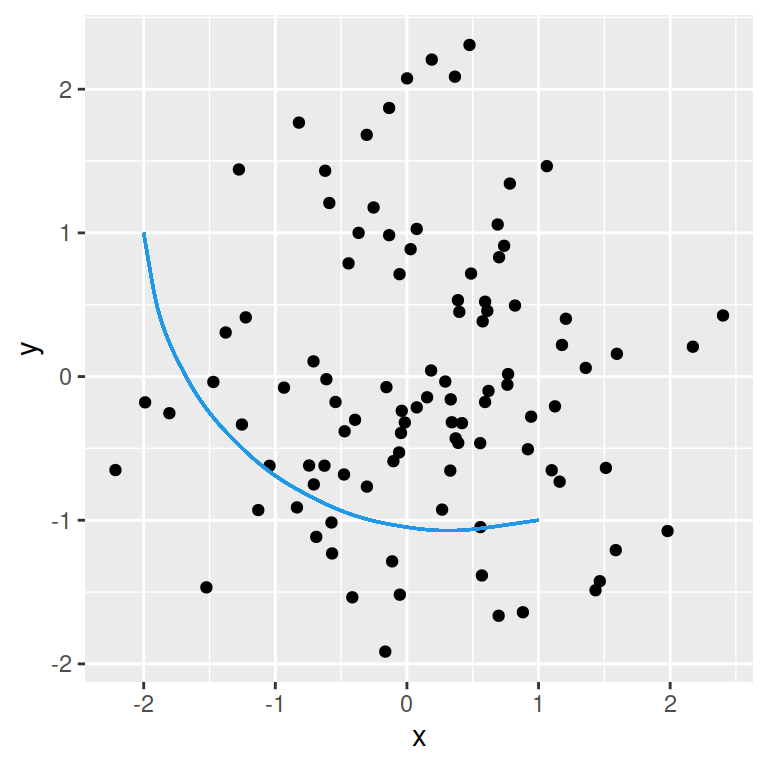Note that you can set the level of curvature passing a value the `curvature` argument of the function. A zero is a straight line, negative values will produce left-handed curves and positive values produce right-handed curves.

``````# install.packages("ggplot2")
library(ggplot2)

# Data
set.seed(1)
df <- data.frame(x = rnorm(100),
y = rnorm(100))

ggplot(df, aes(x = x, y = y)) +
geom_point() +
geom_curve(x = -2, y = 1,
xend = 1, yend = -1,
color = 4,
curvature = -0.2) # Level of curvature``````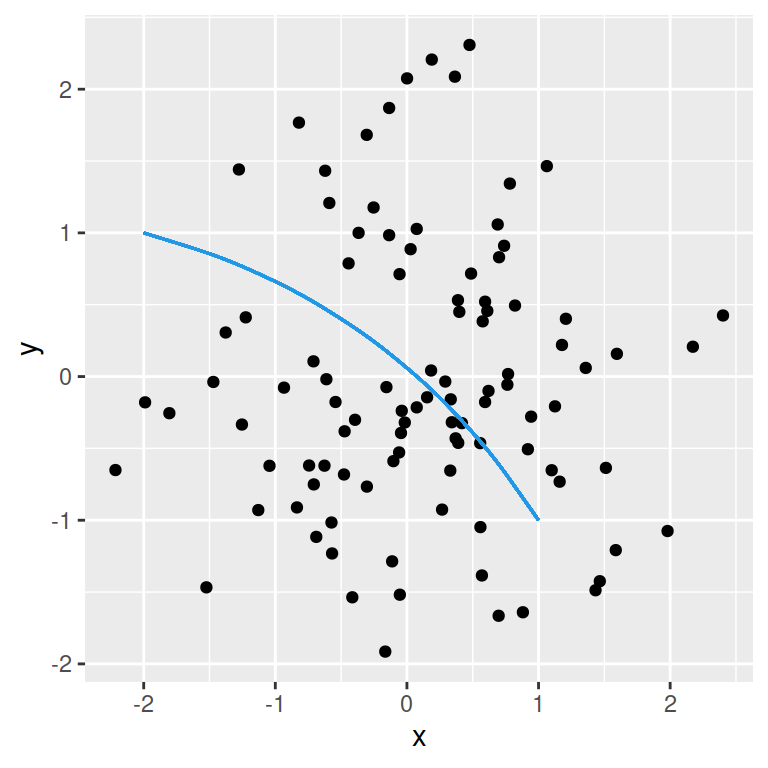Annotate arrow

``````# install.packages("ggplot2")
library(ggplot2)

# Data
set.seed(1)
df <- data.frame(x = rnorm(100),
y = rnorm(100))

ggplot(df, aes(x = x, y = y)) +
geom_point() +
geom_curve(x = -2, y = 1,
xend = 1, yend = -1,
color = 2,
arrow = arrow())``````